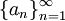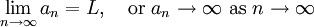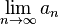# Convergence of Infinite Sequences

Previous: Introduction to Infinite Sequences

Next: Convergence of Infinite Sequences Example

Our next task is to establish, given an infinite sequence, whether or not it converges. Knowing whether or not a given infinite sequence converges requires a definition of convergence.

Definition: Convergence of an Infinite Sequence
Suppose we are given an infinite sequence. This sequence has a limit L, if an approaches L as n approaches infinity. We write this asMoreover, if the number L exists, it is referred to as the limit of the sequence and the sequence is convergent. A sequence that is not convergent is divergent.

The above definition could be made more precise with a more careful definition of a limit, but this would go beyond the scope of what we need. But our definition provides us with a method for testing whether a given infinite sequence converges: if the limittends to a finite number, the sequence converges. Otherwise, it diverges.

Previous: Introduction to Infinite Sequences

Next: Convergence of Infinite Sequences Example